# Chopper - Notes | Study Electrical Engineering SSC JE (Technical) - Electrical Engineering (EE)

 1 Crore+ students have signed up on EduRev. Have you?

CHOPPERS

• Chopper circuit provides adjustable DC from fixed DC. If is analogous’ to the transformer of AC.

It is mainly categorized into two types:

• DC chopper

Principle of Chopper Operation

• It is high speed ON/OFF semiconductor switch (e.g. SCR).
• Inductor L and FWD are used to make load current to be continuous.
• From figure, it can be seen that average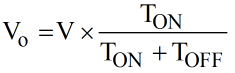where, T = TON + TOFF

f = [1/T] is called as chopping frequency

δ = [TON/T] = duty cycle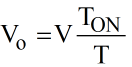•  Form equation it is clear that VO can be controlled either by controlling TON or by controlling f.

Types of Chopper Circuits Classification Of Choppers

Choppers are classified as per the direction voltage and current in five categories,
(a) Class A choppers
(b) Class B chopper
(c) Class C chopper
(d) Class D chopper
(e) Class E chopper.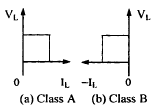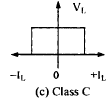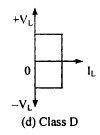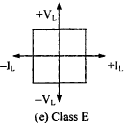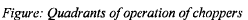Class A Chopper The step down chopper described in sections 5.2, 5.3, 5.4 and 5.5 is class A chopper. The load current II can flow out of the source and into the load, both VI and II. are positive and chopper operates in first quadrant only. Figure (a) shown its quadrant of operation.

Class B chopper It is also a one quadrant chopper but operates in the second quadrant. The load voltage is positive but the load current is negative. Thus the current flows out of load and into the source. Figure (b) shows the quadrant of ‘is operation.

Figure (a) shows the circuit of class B chopper.
Thyristor is shown by switch S. When thyristor is conducting S is closed. When thyristor is off, S is open.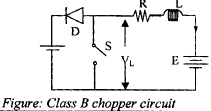When switch is closed, the battery e.m.f. E sends a current through the inductance and the voltage VI. is zero. KVL equation in this mode is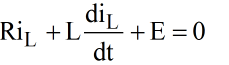Let the current at t = 0 be donated by Imin. Solution of equation with current equal to Imin at t =0 is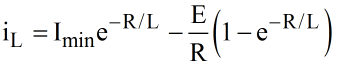This mnode exists for 0 ≤ t ≤ Ton.
Ton can also be written as αT where α is the duty cycle.
When switch S is open, energy stored in inductance is returned to supply through diode D. The current decrease during this mode. If time during this mode is written as t’, KVL equation for this mode is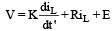Let current at t' = 0 (or t = αT) be denoted by Imax. Solution of equation is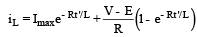This mode exists for 0 ≤ (T–Ton) or for aT ≤ t ≤ T.

Class C chopper

Figure below shows the circuit of class C chopper.
It uses two thyristors shown by switches S1 and S2 and two diodes D1 ,D2. It is also known as two quadrant chopper. The load voltage is always positive.
The current can be either positive or negative. It is a combination of class A and B choppers. ,S1 and Dwork as class A chopper while S2 and D1 work as class B chopper. When S1 or D2 are on, the load current is positive On the other hand when S2 or D1 are on, load current is negative. Power car. flow both from source to ioad and from load to source. It is used in operation and regenerative braking of DC motors.
When motor is driving the load, power into the source.
It should be ensured that both thyrisotrs, S1 and S2 should not be turned on together because this would cause a Short-circuit across the source.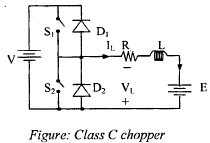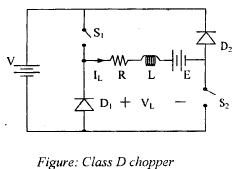Class D chopper Figure shows a class D chopper. The load current is always positive but the load voltage can be positive or negative. This it can operate in first or fourth quadrant. When both S1 and S2 are on, load voltage is positive. If S1 and S2 are off but D1 , D2 conduct, load voltage is negative. In both cases the direction of IL, is the same. When Ton is less than Toff, load voltage is negative. Since VL can be positive or negative, power can flow in both directions i.e. from source to load or from load to source.

Class E chopper A class E chopper can operate in all the four quadrants. Both VL and IL can be positive or negative.
Figure (a) shows the circuit. Two class C choppers when combined give a class E chopper. The operation in the four quadrants is as under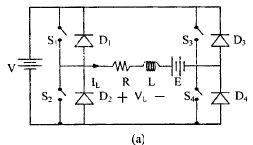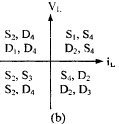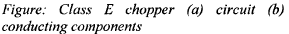Quadrant I: For this operation S4 is on, S2 and Sare off and S1 is operated. When both SS4 conduct, iL flows from source to load. When .S1 is off, iL freewheels through S4 and D2. Thus both v1 and iL are positive and wc get first quadrant operation.

Quadrant II: In this operation S2 is operated while S1 ,S3 and S4 are off. When S2 is on, negative current Hows through L, S2, D4, E. Inductance L stores energy during this current flow. When S2, is off, current is led back to source through D1 and D4. For this feedback of energy,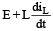should be more than V..

Quadrant III: F or this operation S1 and S4 are off, S2 is on and S3 is operated. When S3 is on, the load is connected to the source through S2, S3 and both vI, and i1, are negative. When S3 is turned off, the negative current freewheels through S2 and D4.

Quadrant IV: S1, S2, S3 are off and S4 is operated. The polarity of e.m.f E has to be reversed for this operation. When is S4, positive current flows through E, S4, D2 L. Energy is stored in inductance L. When .S’4 is turned off, current is fed-back to source through D3 and D2. The load current is positive but load voltage is negative giving operation in fourth quadrant.
The components which conduct are shown in Figure (b).

Thyrsitor Chopper Circuits

The process of opening or turning-off, a conducting thyristor is called commutation. lis dc choppers, ii is essential to provide a separate commutation circuitry to commutate the main power SCR. It may be recalled that a conducting thyristor can be turned, off by reducing its anode current below holding current value and then applying a reverse voltage across the device to enable it to regain its forward blocking capability. T here are several ways of turning-off a thyristor. All these methods differ from

one another in the manner in which commutation is achieved. In DC choppers commutation circuitry has passed through numerous innovations. All these commutation circuits can, however be broadly classified into two groups as under:

1. Forced Commutation: In forced commutation, external elements L and C which do not carry the load current continuously, are used to turn- off a conducting thyristor. Forced commutation can be achieved in the following two ways:

a. Voltage Com m utation : In this scheme, a conducting thyristor is commutated by the application of a pulse of large reverse voltage.
This reverse voltage is usually applied by switching a previously charged capacitor. The sudden application of reverse voltage across the conducting thyristor reduces the anode current to zero rapidly. Then the presence of reverse voltage across the SCPv aids in the completion of its turn-off process (i.e. aids in gaining the forward blocking capability of SCR).

b. Current com m utation: In this scheme, an external pulse of current greater than the load current is passed in the reversed direction through the conducting SCR. When the current pulse attains a value equal to the load current, net pulse current through thyristor becomes zero and the device is turned, off. The current pulses is usually generated by an initially charged capacitor.
An important feature of current commutation is the connection of a diode in anti-parallel with the main thyristor so that voltage drop across the diode reverse biases the main SCR. Since this voltage drop is of the order of 1 volt, the commutation time in current commutation is more as compared to that in voltage commutation.
In both voltage and current commutation schemes, commutation is initiated by getting an auxiliary SCR.

2. Load commutation: In load commutation, a conducting thyristor is turned off when load current flowing through a thyristor either.

a. becomes zero due to the nature of load circuit parameters or

b. is transferred to another device from the conducting thyristor

The document Chopper - Notes | Study Electrical Engineering SSC JE (Technical) - Electrical Engineering (EE) is a part of the Electrical Engineering (EE) Course Electrical Engineering SSC JE (Technical).
All you need of Electrical Engineering (EE) at this link: Electrical Engineering (EE)

## Electrical Engineering SSC JE (Technical)

2 videos|75 docs|37 tests

## Electrical Engineering SSC JE (Technical)

2 videos|75 docs|37 tests

Track your progress, build streaks, highlight & save important lessons and more!

,

,

,

,

,

,

,

,

,

,

,

,

,

,

,

,

,

,

,

,

,

;